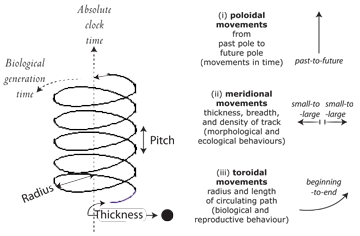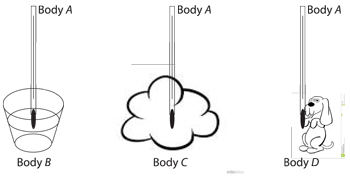# Introduction

Figure 0.10We shall now prove to you:

1. That a population free from Darwinian competition and evolution is impossible.

2. That every population and species can be uniquely determined by three—and only three—properties. We can describe them using the “helicoid” or three dimensional version of a helix shown in Figure 0.10. The three properties are then (a) the pitch, (b) the all-around three-dimensional thickness, and (c) the radius of that helicoid. The pitch is the movement directly up the central axis, which has two poles. The past is one axis pole, the future another. Every population and species therefore moves (i) poloidally or in absolute clock time at some rate. This is always directly vertically from the past pole towards the future one. Every population also engages in behaviours in the surroundings. These are lateral movements in the surroundings that establish the helicoid track's density, energy, density and other three-dimensional properties. These are the movements the population makes (ii) meridionally, i.e. laterally across and about the helicoid and that determine its thickness and texture, as it also moves poloidally, in time. These two sets of activities combine, all around the helicoid, so that the population moves around, as if in a doughnut shape or torus. The population thus moves like a three-dimensional corkscrew through space and time, which are (iii) its set of movements toroidally.

3. We shall further prove to you that the three movements of poloidal, meridional, and toroidal completely determine (a) the genes, (b) the life span, and (c) the entire ecology and behaviour of every possible population and species.

4. That granted the above, every population and species can be uniquely summarized by a single number—its ‘evolutionary potential’—that declares the above three properties, and so that states its entire evolutionary history.

___________________

We will do these things simply, logically, and deductively.

We will even provide you with four equations to make the case.

We will also demonstrate that all biological organisms are subject to the three constraints upon biological populations which we will state. We then further prove that they abide by four laws of biology, and four maxims of ecology, which we will also state.

___________________

Figure 0.11We have to start all this somewhere.

So … we ask you to accept the following ‘common notion’:

Things that are equal to the same thing are also equal to each other.

That common notion is the basis for the proposition that:

If a Body A be in thermal equilibrium with two other bodies, B and C, then B and C are in thermal equilibrium with one another.

The Proposition can be extended to embrace many other bodies. As we can see in Figure 0.11, the Body A it refers to is, of course, a thermometer. It also really doesn't matter how different the various bodies are. If the readings we take on our thermometer for Bodies B, C, and D match each other, then they are all at the same temperature as our thermometer or our Body A. This is a fundamental fact of life and one of the four laws of thermodynamics (sometimes called the zeroth law, sometimes also called the fourth law).

And that's about all we need to prove that a population free from Darwinian evolution is impossible.

If you accept the above common notion and the zeroth law derived from it, then the four laws, four maxims, and three constraints will inevitably follow. The job is done.

___________________

Oh! … one last thing!! We also ask you to accept the existence and power of gravity.

___________________

So … let's get started so you can see for yourself how straight forward it all is.